## Main

Electron–phonon coupling in SWNTs is usually studied using Raman spectroscopy; this technique is useful for investigating ground-state vibrations14, whereas photoexcited-state vibrational dynamics remain largely unknown because, in the frequency domain, phonon replicas are hardly detectable in the presence of substantial inhomogeneous broadening. Time-domain observation of phonon dynamics has much lower sensitivity with respect to conventional Raman, but it enables direct measurement of excited-state dynamics, vibrational dephasing and mode coupling in a distinct way15,16. Coherent phonon detection allows resolution in time of wavepacket dynamics that is otherwise averaged-out in standard Raman scattering.

To detect coherent phonons in SWNTs, we use a standard pump–probe configuration, in which the observed quantity is the modulation depth in the differential transmission17T/T); details of the experimental setup are provided in the Methods section. Figure 1a shows ΔT/T dynamics of SWNTs grown by the high-pressure carbon monoxide procedure dispersed in polymethylmethacrylate films following excitation with a sub-10-fs visible pulse (1.8–2.4 eV bandwidth), probed at an energy of 2.1 eV. The signal exhibits an initial photobleaching, which quickly turns into photoinduced absorption (PA). The fast photobleaching decay is ascribed to relaxation of the higher-lying exciton (second in an increasing energy scale) to the lower one, taking place with a 40-fs time constant18. The PA signal is generated by this lower exciton4,5 and decays on the ps timescale, in agreement with previous results19,20,21. As shown in Fig. 1a, there is a clear oscillation in the ΔT/T amplitude. The Fourier transform (FT) of the oscillatory component (Fig. 2a) shows a strong peak at 252 cm−1 (132-fs period). This frequency can be recognized as the RBM, associated with expansion and contraction of the tube cross-section14. The observation is related to an ensemble of semiconducting SWNTs, with diameters of about 0.95 nm, all vibrating in phase. A similar result is obtained pumping with a 30-fs pulse in the near-infrared (0.92 eV) range and probing in the visible (2 eV) range with a sub-10-fs pulse (Fig. 1b). Here, the lowest exciton is directly excited and its PA rises promptly; a clear modulation at the frequency of 259 cm−1 is visible on top of it.

The 'displacive excitation' mechanism22 can account for coherent phonon generation in SWNTs: carbon atoms do not have time to move during the short pulse excitation. However, in the excited-state electronic configuration, they may have a different equilibrium position. This will occur only for those modes that do not distort the molecular symmetry during vibration, namely totally symmetric modes (those observed in optical transitions and resonance Raman scattering). Calculations based on a semi-empirical excited-state molecular dynamics (ESMD) approach13 confirm that both the RBM and G modes have substantial coupling with the electronic transitions. Calculated dimensionless displacements from the ground state to the lowest optically active exciton state are 0.2 for the RBM and 0.4 for the G mode. As a consequence, the atoms oscillate along the selected configurational coordinate minimum. Unless coherence is destroyed by intra- and/or inter-tube perturbations, the vibrational motion of the ensemble is that of a single-molecule trajectory. All of the nanotubes involved breathe in phase, by periodically modulating their diameter. We find that the semiconducting nanotubes which can be excited in our experiments are (10,3), (9,5), (9,4) and (7,6) with RBM frequencies 253, 242, 257 and 263 cm−1 in the ground state and the second exciton resonance at 1.95, 1.85, 2.03 and 1.92 eV, respectively23. This justifies the observation of oscillations with frequencies around 250 cm−1. The lack of RBM modes at 200 cm−1 indicates that coherence is not generated in metallic tubes, although they are present in our sample. The modulation depth decays slowly, with time constant T2≈1.6 ps, allowing more than 20 oscillations to be detected. Analogous to nanoelectromechanical systems24, this phenomenon suggests that SWNTs can be light-driven nanoscale oscillators, behaving as molecular springs at 7.5 THz frequency, actuated by an optical pulse. Our results demonstrate that coherent control of structural modifications in SWNTs is indeed feasible25.

The inset of Fig. 1a shows a portion of the ΔT/T trace acquired with a higher sampling rate (2.66-fs steps) and indicates that there is also modulation at high frequency, corresponding, in the FT spectrum, to 1,588 cm−1 (21-fs period). This value agrees with that of the G band assigned to the totally symmetric longitudinal carbon-stretching mode, as shown in the comparison with the Raman spectrum (Fig. 2b).

A further tool available for analysing our data is the sliding window FT26 (SWFT), which yields instantaneous frequencies and amplitudes of the molecular vibrations. In Fig. 3a the SWFT corresponding to the trace in the inset of Fig. 1a is reported as a contour plot, clearly showing that there is a modulation in the G-mode frequency. The modulation period can be extracted by carrying out a FT of the time-dependent band peak position. The result of such an operation, shown in Fig. 3b, is striking: the modulation frequency coincides with that of the RBM. A straightforward interpretation can be given: the breathing SWNT sample passes through different geometries, corresponding to different potential energy curvatures for the G mode. The large period difference between the two modes (τRBM/τG=130/21≈6) leads to decoupling of the oscillations in the time domain so that the high-frequency mode follows the low-frequency mode adiabatically. Artefacts resulting from an overlap between the broadened FT peaks in the SWFT spectra can be disregarded because of such dynamical separation17. Frequency modulation should have a corresponding signature in the frequency domain, where sidebands are expected; accordingly, the experimental FT spectrum in the whole time range (solid line in Fig. 2a, inset), contains small peaks that do not correspond to any feature in the Raman spectrum and are almost equally spaced in wavenumbers, at 1,078, 1,335, 1,841 and 2,104 cm−1, their difference being approximately that of the RBM. Note that an amplitude modulation would also lead to sidebands in the frequency domain, however with a different phase relation; thus, the critical check for distinguishing the two is the time-domain SWFT. The sidebands do not appear as in Raman spectra, where only some combination modes can be observed27. However, such bands are extremely small, and they would be subjected to ultrafast phase loss, so they could not be due to G and RBM mode coupling, that would in any case occur in the ground electronic state. As a consequence those would not give rise to any signal in the time domain. On the contrary, our experimental results suggest that the G-mode frequency depends on the RBM configurational coordinate, ωG=ωG(QRBM(t)), where t is time. This dependence can easily be modelled by assuming QRBM(t)=Q0cos(ωRBMt) and expanding to the second order, obtaining: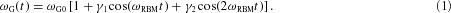Here ωG0 is the unperturbed G-mode frequency, and γ1 and γ2 are the expansion coefficients representing coupling constants with the fundamental and second harmonic of the RBM mode. Using γ1=0.156 and γ2=0.094 we can reproduce both the FT spectrum (circles in Fig. 2a, inset) and the spectrogram. Note that a qualitative justification of the above assumption can also be derived by the two-coupled classic oscillator model (see the Methods section).Figure 3: Analysis of the frequency modulation of the G mode.

To gain insight into the effective nonlinear phonon coupling mechanism, we carried out modelling based on the ESMD approach. All reported computational results are for the chiral (7,6) tube with finite boundaries, but sufficiently long (5 nm) to mimic the infinite tube limit and exclude edge effects from the tube ends. The simulated photoexcited trajectories have been propagated along all nuclear degrees of freedom for up to 1 ps. These calculations assume the Born–Oppenheimer approximation: nuclei are classical and electrons are quantum. No approximations to the vibrational couplings and anharmonicities were made because the underlying hamiltonian (Austin Model 1, AM1, ref. 28) has these effects incorporated in the dependence of its matrix elements on the nuclei positions. The RBM and G mode are both Raman-active Frank–Condon vibrations, that is, their equilibrium positions change on electronic excitation. However, tube-diameter modification in going from the ground to the first exciton state is not simple. In Fig. 4a, we show the change in tube radius d r with atom coordinate along tube Z axis calculated for the (7,6) tube shown in the inset. Overall, relaxation of the photoexcitation results in an increase in the average diameter in the middle of the tube, causing it to bulge. This reflects an exciton self-trapping phenomenon similar to that observed in conjugated polymers13. Surprisingly, there is also a corrugation of the tube surface in the excited state, which clearly leads, in support of our conjecture, to coupling of radial and longitudinal modes.

We also calculated ground state normal modes for the (7,6) tube and obtained 323 and 1,737 cm−1 as RBM and G-mode frequencies, respectively. These are overestimated compared with the experiment, which is typical for AM1. From the experiment, it is possible to estimate the anharmonic coupling strength between the RBM and G mode in the excited state to be about 2 cm−1. However, calculated ground-state cubic anharmonicities do not exceed 0.05 cm−1 and quartic anharmonicities do not exceed 0.01 cm−1; that is, radial and longitudinal modes are essentially uncoupled in the ground state. Direct calculation of vibrational normal modes and anharmonicities in the excited state is not possible due to the computational expense. Using ground-state normal modes as a basis, we numerically estimated frequencies of the RBM and G mode in the excited state to be 324 and 1,727 cm−1, respectively. Because of the corrugation of the tube surface in the excited state, these modes weakly mix (Duschinsky rotation), which we estimate, from the off-diagonal elements of the hessian matrix, to be about 1%. Finally, to explore the anharmonic coupling between RBM and G mode in the excited state, we ran molecular dynamics simulations. The trajectories were started from ground- (excited-) state equilibrium geometries with the G mode excited by two vibrational quanta (0.43 eV). In the ground state, the trajectories (not shown) are perfectly harmonic with all vibrational energy preserved in the G mode on the ps timescale. The situation is very different in the excited state. Figure 4b shows the FT of a 1 ps trajectory of the RBM dimensionless displacement. In addition to the fundamental RBM frequency, we observe a weak component of the excited state G-mode frequency appearing due to linear mixing of the G and RBM in the excited state. Furthermore, the G-mode component in the FT spectrum (inset of Fig. 4b) contains equally spaced small satellites that correspond to respective overtones of the RBM mode, strikingly similar to Fig. 2a. This provides computational confirmation of anharmonic coupling between radial and longitudinal modes induced in the excited state.

In summary, this study shows that ultrashort light pulses can drive coherent atomic motion in SWNTs, in spite of the large inhomogeneous broadening. We have demonstrated the high selectivity of the mechanism: metallic tubes are not involved; essentially only two modes in semiconducting tubes are excited. With such a technique, nonlinear molecular dynamics can be observed. The exciton state modulates the phonon field introducing the nonlinearity, which manifests itself as vibrational coupling. Quantum-chemical dynamic computations show that on excitation, the nanotube's surface becomes locally distorted, inducing a coupling between the radial and longitudinal modes, which gives rise to the frequency modulation experimentally observed in the time domain.

## Methods

### Ultrafast spectroscopy

The ultrafast spectroscopy setup is based on a non-collinear optical parametric amplifier (NOPA), pumped by an amplified Ti:sapphire laser (500 μJ, 150 fs, 1 kHz, 780 nm). In the NOPA, a fraction of 780-nm light is focused on a thin sapphire plate to generate a white-light continuum, whereas the remainder is frequency doubled to 390 nm; parametric amplification of the white light takes place in a β-barium borate crystal, pumped by the 390-nm beam, using a suitable non-collinear geometry that guarantees a broad gain bandwidth. The NOPA generates ultrabroadband visible pulses, with a spectrum spanning the 1.8–2.4 eV frequency range. These pulses are then compressed to a nearly transform-limited 6–7 fs duration by multiple reflections onto specially designed chirped dielectric mirrors. The trace in Fig. 1b is obtained by generating the pump pulses with a second NOPA similar to that described above, but tuned to the near-infrared (0.92 eV) region.

Pump–probe experiments are carried out in a standard non-collinear configuration using only reflective optics. Time-resolved measurements at specific wavelengths are obtained by spectrally filtering, with 10-nm bandwidth interference filters, the probe beam after passing through the sample and combining differential detection with lock-in amplification. All measurements are carried out in air at room temperature.

### Sample preparation

We studied samples of SWNTs grown by the high-pressure carbon monoxide procedure. After purification, buckytubes (Carbon Nanotechnologies) were functionalized with poly(ethylene glycol) chains to improve dispersion in a polymer matrix. The derivatization was achieved through amidation of nanotube-bound carboxylic acids with poly(ethylene glycol)-amine29. The functionalized SWNTs were embedded in polymethylmethacrylate films that were cast on glass cover slips. Resonance Raman spectra showed that the oxidation reaction used for the functionalization destroyed the nanotubes with diameters below ≈0.85 nm (ref. 30).

### Data analysis

The FTs are carried out on the oscillatory component of the signal, after subtracting a slowly varying background. The spectrograms are obtained by carrying out a SWFT, that is, a series of FTs carried out on different time windows with position swept along the whole measurement domain. The SWFT is obtained according to the formulawhere s(t) is the oscillatory component of the signal and g(tτ)=exp[−(tτ)n/τPn] is a super-gaussian window function. In our calculation we used n=6 and τp=70 fs.

### Dynamics of coupled classical oscillators

To illustrate the model used for experimental fitting (equation (1)) based on the parametric dependence of the G-mode frequency on the RBM configurational coordinate, we consider a very simple model of the dynamics of two coupled anharmonic classical oscillators. The general form of the system lagrangian in the normal coordinate representation qi isHere, terms higher than quartic anharmonicities ki j n m have been truncated. By denoting q1=q as the coordinate of the G mode with mass m1=m, spring constant k1=k and bare frequency, and q2=Q being the coordinate of the RBM with mass m2=M, spring constant k2=K and frequency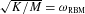and applying the Lagrange equation, we obtain nonlinear equations of motion for the two oscillators. We can write the following equations considering, in particular, the lower-order terms which allow a description of the interaction between the two oscillators:where α=2k122/M and β=2k112/m, σ=2k1122/m, and η=2k1122/M.

According to the model we are proposing, where the Q mode is a slowly varying potential for the higher frequency q mode, we can assume Q(t)cos(ωRBMt). Therefore, for the q mode we can write: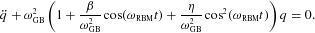An expansion of the oscillator frequency in powers of β and η up to the linear terms,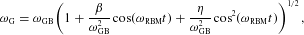gives the following expression for modulated G-mode frequency in the form of equation (1):with the renormalized fundamental frequencyand the modulation parameters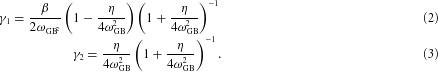Equation (2a) clearly show that the value of the modulation parameters γ1 and γ2 is a result of the interplay between the values of the cubic and quartic anharmonicities entering through β and η. Accordingly, their small but close values obtained in the paper from the fit of the pump–probe experiment and also confirmed by the numerical simulations, clearly indicate that both anharmonic terms are equally responsible for the mode coupling.

### Signatures of the excited-state mode coupling in the pump–probe response

The pump–probe signal induced by the ultrafast non-overlapping pulses can be analysed using the doorway–window representation, which for a vibrationally coupled two-level electronic system reads31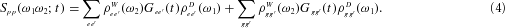Each component in equation (3) is a product of three terms. First, the doorway function ρe eD(ω1) [ρg gD(ω1)] describing the excitation of the vibrational coherence |e〉〈e′|[|g〉〈g′|] in the excited [ground] electronic state by the pump pulse. Its dependence on ω1 mimics the optical absorption lineshape at experimental temperature. Second, Ge e(t)[Gg g(t)] is the Green function describing time evolution of the vibrational coherence |e〉〈e′|[|g〉〈g′|] during the delay time t between the pump and probe pulses. Finally, ρe eW(ω2)[ρg gW(ω2)] is the window function having the shape of the optical absorption band at infinite temperature, and describing the effect of the probe pulse absorption. If the pump (probe) pulse bandwidth is narrower than the absorption bandwidths it should be convoluted with the doorway (window) functions.

The first term in equation (3) depends on Ge e(t), and, therefore, carries spectroscopic signatures of the vibrational dynamics in the excited electronic state. The second one, containing Gg g(t), represents the ground-state vibrational dynamics, and carries similar information on the mode coupling as Raman spectra. As a result, in the signal analysis we should focus on the first term. To identify the features observed in Fig. 1 and in the inset of Fig. 2a, we follow the assumption that the RBM and G-mode dynamics are classical. Then, the corresponding Green function is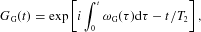where ωG(t)=ωG0[1+γ1cos(ωRBMt)+γ2cos(2ωRBMt)] is the G-mode frequency adiabatically modulated by the RBM mode, and T2 is the dephasing time. The integral can be easily evaluated givingFurther expanding the exponential in powers of γ1 and γ2, and retaining terms up to the linear one, the Green function can be recast as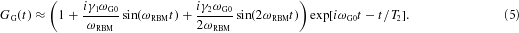This expression can be used to analyse the observed time-domain signal in Fig. 1.

To gain insight into the features present in the inset of Fig. 2a, the FT of equation (4) on the interval of t= () has to be made, transforming the Green function to the following frequency-domain representation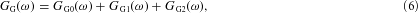where the terms in the sum are resonant at ωG0, ωG0±ωRBM, and ωG0±2ωRBM respectively. By introducing the homogeneous line width δ=2π/T2, below, we provide expressions for these terms. The main peak at ω=ωG0 is described by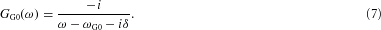The first sidebands ω=ωG0±ωRBM appear in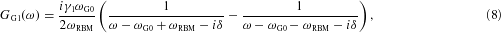and finally, the second sidebands ω=ωG0±2ωRBM are given by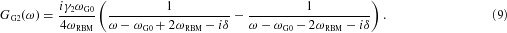An important observation in equations (5)–(8) regarding the ratio of the peaks' absolute values can be made. Provided the doorway and window functions are flat in the spectral region considered and the line widths of the sidebands are identical, the ratio of the fundamental ωG0, first ωG0±ωRBM, and the second ωG0±2ωRBM sidebands is proportional to the 1:γ1:γ2 ratio. As shown above, γ1 and γ2 are proportional to the anharmonic coupling constants, and therefore, the sideband intensities carry direct information on the mode coupling constants.

### Quantum chemical calculations

We used the Austin Model 1 (AM1) hamiltonian28 and the ESMD computational package13 to follow photoexcited adiabatic dynamics on ps timescales for all calculations presented in this letter. The ESMD approach calculates the excited-state potential energy as Ee(q)=Eg(q)+Ω(q) in the space of all nuclear coordinates q which span the entire 3N−6-dimensional space, N being the total number of atoms in the molecule. Here Ω(q) is an electronic transition frequency to the lowest optically active excited state of the molecule calculated using the time-dependent Hartree–Fock approximation. The program uses efficient analytic derivatives of Ee(q) with respect to each nuclear coordinate qi to calculate forces and subsequently to step along the excited-state hypersurface using these gradients. A standard Verlet molecular dynamics algorithm has been used for propagation of the Newtonian equations of motion along all (i=1,…,3N−6) nuclear degrees of freedom qi. If no dissipative processes are included, the total molecular energy Ee(q) is conserved. Subsequent analysis of the photoexcited trajectory in Fourier space allows us to identify periods of participating vibrational motions. Inclusion of an artificial dissipative force into the ESMD equations of motion allows us to determine the minimum of the excited-state potential energy surface Ee(q) corresponding to the relaxed geometry.